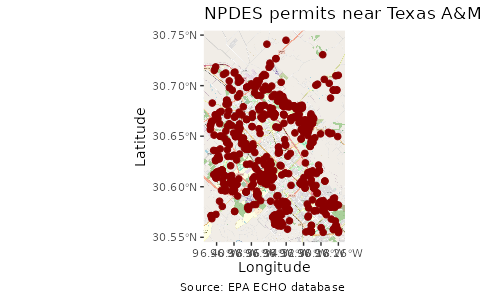echor can also return spatial data frames known as simple features (https://r-spatial.github.io/sf/), to facilitate creation of maps. Both echoAirGetFacilityInfo and echoWaterGetFacilityInfo include arguments to return simple feature dataframes.

Using sf, ggplot, and ggspatial we can quickly create a map of downloaded data.


library(echor)
library(ggplot2)
library(ggspatial)
library(dplyr)
library(sf)

df <- echoWaterGetFacilityInfo(xmin = '-96.407563', ymin = '30.554395',
xmax = '-96.25947', ymax = '30.751984',
output = 'sf')

## Make the map
ggplot(df) +
annotation_map_tile(zoomin = 0, progress = "none") +
geom_sf(inherit.aes = FALSE, shape = 21,
color = "darkred", fill = "darkred",
size = 2) +
labs(x = "Longitude", y = "Latitude",
title = "NPDES permits near Texas A&M",
caption = "Source: EPA ECHO database")